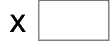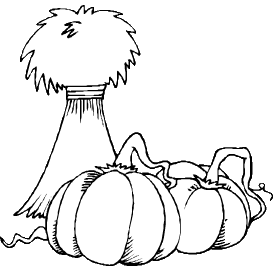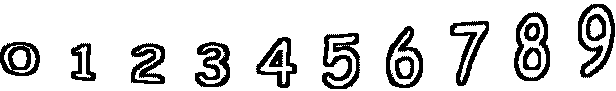8= 800   78= 132699= 8019   8= 224   95= 8930   69= 2967 95= 3610   63= 5670   54= 4752   61= 4209